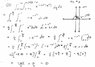# Double integrate from cartesian to polar and then evaluated

• jimbo71

## Homework Statement

convert double integral from line one to polar integral and then evaluate
see problem 12 attachment

y=rsinx
x=rcosx
r^2=x^2+y^2

## The Attempt at a Solution

see problem 12 attachment
I calculate a area of zero. are my limits wrong and if so which ones, or did i make a mistake in the aritmatic, or something else?

#### Attachments

•problem 12.jpg
29.8 KB · Views: 390
You start to go wrong at the end of the 5th line. $e^{-1}$ does not equal $e$.